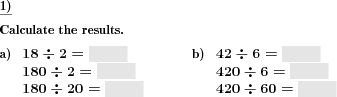Custom math worksheets at your fingertips# Details for problem "Division series 1x1 with factor 10"

Quickname: 8345

Elementary School, Primary School, Middle School.

## Summary

Division problem series with divisor and dividend alternating with factor ten.

## Example## Description

Each problem is a series of three division tasks with the basis being the times tables.

Two factors f1 and f2 and their product p=f1*f2 are being determined. These numbers are used to construct three quotients:

```p / f1 =      e.g.  42 / 6 =
10p / f1 =    e.g.  420 / 6 =
10p / 10f1 =  e.g.  420 / 60 =
```

Each of these is presented to be solved.

It can be chosen whether they will always be posed in this order, in reverse or in random order.

A catch question may be included in a random problem, where f1 and f2 will be swapped, so that instead of 420 / 7, the problem will ask for 420 / 6.

Download free printable worksheets for this math problem here. The worksheet contains the problems only, the solution sheet includes the answers. Just click on the respective link.

•Worksheet 1Solution sheet with answers
•Worksheet 2Solution sheet with answers
•Worksheet 3Solution sheet with answers

If you can not see the solution sheets for download, they may be filtered out by an ad blocker that you may have installed. If this is the case, please allow ads for this page and reload the page. The solution sheets will then reappear.

• Do these sample worksheets do not really fit?
• Do you need more math worksheets, with a different level of difficulty?
• Would you like to combine different problems on a worksheet and adjust them to your needs?
• As a teacher, you can put together your own worksheets using the automatically generated math problems provided.
With a free initial credit, you can start creating your own math worksheets in a few minutes.

You can try it for free! Register here, to create custom worksheets now!

## Customization options for this problem

Parameter
Possible values
Number of problems
1, 2, 3, 4, 5, 6, 7, 8, 9, 10
Order of series
random, ascending, descending
Catch question
Yes, No

## Similar problems

Remark
Description
Simple division problems from the times table
Division problems from the multiplication tables
As a series of multiplication problems
Multiplication problem series introducing factor of ten